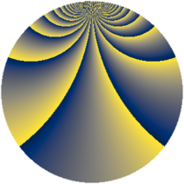# Properties

 Label 1792.2.bvLevel $1792$ Weight $2$ Character orbit 1792.bv Rep. character $\chi_{1792}(27,\cdot)$ Character field $\Q(\zeta_{64})$ Dimension $8128$ Sturm bound $512$

# Learn more

## Defining parameters

 Level: $$N$$ $$=$$ $$1792 = 2^{8} \cdot 7$$ Weight: $$k$$ $$=$$ $$2$$ Character orbit: $$[\chi]$$ $$=$$ 1792.bv (of order $$64$$ and degree $$32$$) Character conductor: $$\operatorname{cond}(\chi)$$ $$=$$ $$1792$$ Character field: $$\Q(\zeta_{64})$$ Sturm bound: $$512$$

## Dimensions

The following table gives the dimensions of various subspaces of $$M_{2}(1792, [\chi])$$.

Total New Old
Modular forms 8256 8256 0
Cusp forms 8128 8128 0
Eisenstein series 128 128 0

## Trace form

 $$8128q - 64q^{2} - 64q^{4} - 32q^{7} - 64q^{8} - 64q^{9} + O(q^{10})$$ $$8128q - 64q^{2} - 64q^{4} - 32q^{7} - 64q^{8} - 64q^{9} - 64q^{11} - 32q^{14} - 64q^{15} - 64q^{16} - 64q^{18} - 32q^{21} - 64q^{22} - 64q^{23} - 64q^{25} - 32q^{28} - 64q^{29} - 64q^{30} - 64q^{32} - 32q^{35} - 64q^{36} - 64q^{37} - 64q^{39} - 32q^{42} - 64q^{43} - 64q^{44} - 64q^{46} - 32q^{49} - 64q^{50} - 64q^{51} - 64q^{53} - 32q^{56} - 64q^{57} - 64q^{58} - 64q^{60} - 32q^{63} - 64q^{64} - 64q^{65} - 64q^{67} - 32q^{70} - 64q^{71} - 64q^{72} - 64q^{74} - 32q^{77} - 64q^{78} - 64q^{79} - 64q^{81} - 32q^{84} - 64q^{85} - 64q^{86} - 64q^{88} - 32q^{91} - 64q^{92} - 64q^{93} - 64q^{95} - 32q^{98} - 64q^{99} + O(q^{100})$$

## Decomposition of $$S_{2}^{\mathrm{new}}(1792, [\chi])$$ into newform subspaces

The newforms in this space have not yet been added to the LMFDB.